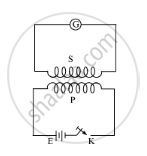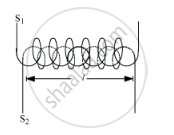Share

# Consider Two Concentric Circular Coils, One of Radius R1 and the Other of Radius R2 (R1 < R2) Placed Coaxially with Centres Coinciding with Each Other. Obtain the Expression for the Mutual Inductance of the Arrangement. - CBSE (Science) Class 12 - Physics

#### Question

Consider two concentric circular coils, one of radius r1 and the other of radius r2 (r1 < r2) placed coaxially with centers coinciding with each other. Obtain the expression for the mutual inductance of the arrangement.

#### SolutionCoefficient of mutual induction − consider two coils and S. Suppose that a current I is flowing through the coil P at any instant i.e.,

Φ ∝ I

Φ = MI… (i)

If ‘e’ is the induced emf produced in the S-coil, then

e=(dphi)/dt=-d/dt(MI)=-M(dl)/dt

Mutual Inductance of two concentric coils, one of radius r1 and the other of radius r2 (r1 < r2) placed coaxially with centers coinciding with each other:Consider two circular coil S1 and S2 of same length l, such that coil S2 surrounds coil S1 completely

Let

n1 − Number of turns per unit length of S1

n2 − Number of turns per unit length of S2

I1 − Current passed through solenoid S1

Φ21 − Flux linked with S2 due to current flowing through S1

Φ21 ∝ I1

Φ21 = M21I1

Where M21 is the coefficient of mutual induction of the two coils

When current is passed through S1, an emf is induced in S2.

Magnetic field produced inside  S1 on passing current through it,

B1 = μ0n1I1

Magnetic flux linked with each turn of Swill be equal to B1 times the area of the cross-section of S1.

Magnetic flux linked with each turn of the  S2 = B1A

Therefore, total magnetic flux linked with the S2,

Φ21 = B1A × n2l = μ0n1I1 × A× n2l

Φ21 = μ0n1n2AlI1

∴ M21 = μ0n1n2Al

Similarly, the mutual inductance between the two coils, when current is passed through coil S2 and induced emf is produced in coil S1, is given by

M12 = μ0n1n2Al

∴M12 = M21 = M (say)

Hence, coefficient of mutual induction between the two coil will be

 M=mu_0n_1n_2Al
Is there an error in this question or solution?

#### Video TutorialsVIEW ALL 

Solution Consider Two Concentric Circular Coils, One of Radius R1 and the Other of Radius R2 (R1 < R2) Placed Coaxially with Centres Coinciding with Each Other. Obtain the Expression for the Mutual Inductance of the Arrangement. Concept: Inductance - Mutual Inductance.
S